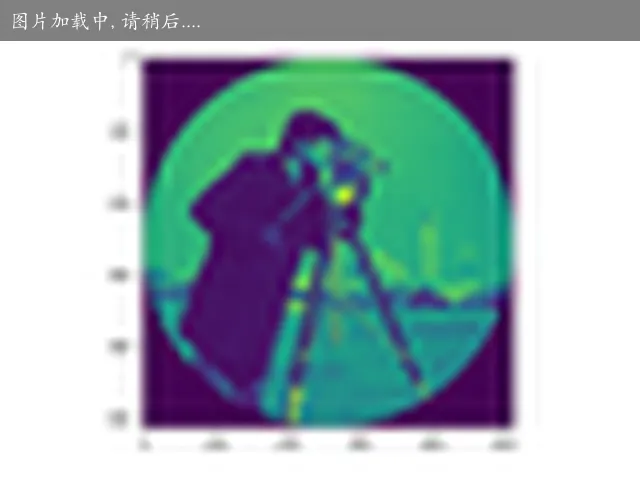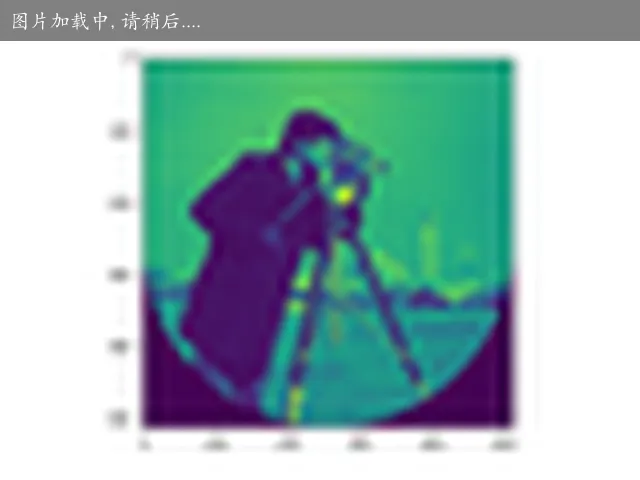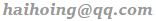## NumPy 切片和索引

``````import numpy as np

a = np.arange(10)
b = a[2:7:2] # 从索引 2 开始到索引 7 停止，间隔为 2
print(b)
``````
``````[2  4  6]
``````

``````import numpy as np

a = np.array([[1,2,3],[3,4,5],[4,5,6]])
print (a[...,1])   # 第 2 列元素
print (a[1,...])   # 第 2 行元素
print (a[...,1:])  # 第 2 列及剩下的所有元素
``````
``````[2 4 5]
[3 4 5]
[[2 3]
[4 5]
[5 6]]
``````
``````>>> import numpy as np
>>> a = np.array([[[1,2],], [[3,4]]])
>>> a
array([[[1, 2]],
[[3, 4]]])

>>> a[..., :1] # an index can only have a single ellipsis ('...')
array([[],
[]])

>>> a[:, :, :1]
array([[],
[]])
``````

## NumPy 高级索引

### 整数数组索引

``````import numpy as np

x = np.array([[1,  2],  [3,  4],  [5,  6]])
y = x[[0,1,2],  [0,1,0]]
print (y)
``````
``````[1  4  5]
``````

``````import numpy as np

x = np.array([[  0,  1,  2],[  3,  4,  5],[  6,  7,  8],[  9,  10,  11]])
print ('我们的数组是：' )
print (x)
print ('\n')
rows = np.array([[0,0],[3,3]])
cols = np.array([[0,2],[0,2]])
y = x[rows, cols]
print ('这个数组的四个角元素是：')
print (y)
``````
``````我们的数组是：
[[ 0  1  2]
[ 3  4  5]
[ 6  7  8]
[ 9 10 11]]

[[ 0  2]
[ 9 11]]
``````

``````import numpy as np

a = np.array([[1,2,3], [4,5,6], [7,8,9]])
b = a[1:3, 1:3]
c = a[1:3, [1,2]]
d = a[..., 1:]
print(b)
print(c)
print(d)
``````
``````[[5 6]
[8 9]]

[[5 6]
[8 9]]

[[2 3]
[5 6]
[8 9]]
``````

### 布尔索引

``````import numpy as np

x = np.array([[  0,  1,  2],[  3,  4,  5],[  6,  7,  8],[  9,  10,  11]])
print ('我们的数组是：')
print (x)
print ('\n')
# 现在我们会打印出大于 5 的元素
print ('大于 5 的元素是：')
print (x[x > 5])
``````
``````我们的数组是：
[[ 0  1  2]
[ 3  4  5]
[ 6  7  8]
[ 9 10 11]]

[ 6  7  8  9 10 11]
``````

``````import numpy as np

a = np.array([np.nan, 1, 2, np.nan, 3, 4, 5])
print (a[~np.isnan(a)])
``````
``````[1. 2. 3. 4. 5.]
``````

``````import numpy as np

a = np.array([1,  2+6j,  5,  3.5+5j])
print (a[np.iscomplex(a)])
``````
``````[2.+6.j 3.5+5.j]
``````

### 花式索引

#### 传入顺序索引数组

``````import numpy as np

x = np.arange(32).reshape((8,4))
print (x[[4,2,1,7]])
``````
``````array([[ 0,  1,  2,  3],
[ 4,  5,  6,  7],
[ 8,  9, 10, 11],
[12, 13, 14, 15],
[16, 17, 18, 19],
[20, 21, 22, 23],
[24, 25, 26, 27],
[28, 29, 30, 31]])

[[16 17 18 19]
[ 8  9 10 11]
[ 4  5  6  7]
[28 29 30 31]]
``````

#### 传入倒序索引数组

``````import numpy as np

x = np.arange(32).reshape((8,4))
print (x[[-4,-2,-1,-7]])
``````
``````[[16 17 18 19]
[24 25 26 27]
[28 29 30 31]
[ 4  5  6  7]]
``````

#### 传入多个索引数组（要使用 np.ix_）

``````import numpy as np

x = np.arange(32).reshape((8,4))
print (x[np.ix_([1,5,7,2],[0,3,1,2])])
``````
``````x[1,0] x[1,3] x[1,1] x[1,2]
x[5,0] x[5,3] x[5,1] x[5,2]
x[7,0] x[7,3] x[7,1] x[7,2]
x[2,0] x[2,3] x[2,1] x[2,2]

[[ 4  7  5  6]
[20 23 21 22]
[28 31 29 30]
[ 8 11  9 10]]
``````

### sample

``````nrows, ncols = camera.shape
row, col = np.ogrid[:nrows, :ncols]
cnt_row, cnt_col = nrows / 2, ncols / 2
outer_disk_mask = ((row - cnt_row)**2 + (col - cnt_col)**2 >
(nrows / 2)**2)
imgshow(camera)
````````````lower_half = row > cnt_row
camera = data.camera()
camera[lower_half_disk] = 0
imgshow(camera)
``````## 向量和矩阵的点乘和叉乘

### 向量

``````import numpy as np
a = np.array([1, 2, 3])
b = np.array([2, 2, 3])
np.dot(a, b)
np.cross(a, b)
a * b
np.multiply(a, b)
``````
``````15 # dot
array([ 0,  3, -2]) # cross
array([2, 4, 9]) # *
array([2, 4, 9]) # multiply
``````

### 矩阵

``````a = np.array([[1,2],[3,4]])
b = np.array([[5,6],[7,8]])
a * b
np.dot(a, b)
np.cross(a, b)
np.multiply(a, b)
``````
``````array([[ 5, 12], # *
[21, 32]])
array([[19, 22], # dot
[43, 50]])
array([-4, -4]) # cross 不知道是个啥
array([[ 5, 12], # multiply
[21, 32]])
``````

### 比较

numpy 库的对象有数组和矩阵，两者看起来长得差不多，但在性质、运算上有很大不同。可通过 array 函数和 mat 函数相互转化。

#### dot 函数

• 对于秩为 1 的数组，执行对应位置相乘，然后再相加，等价于向量的点乘；
• 对于秩不为 1 的二维数组，执行矩阵乘法运算，等价于矩阵的叉乘；

#### multiply 函数

• 数组和矩阵对应位置相乘，输出与相乘数组 / 矩阵的大小一致，效果上与运算符 * 对数组效果一样。

#### 运算符 * 号

• 对数组执行对应位置相乘，等价于 multiply 函数；
• 对矩阵执行对应位置相乘，等价于 multiply 函数。

## 参考

If you have any questions or feedback, please reach out.